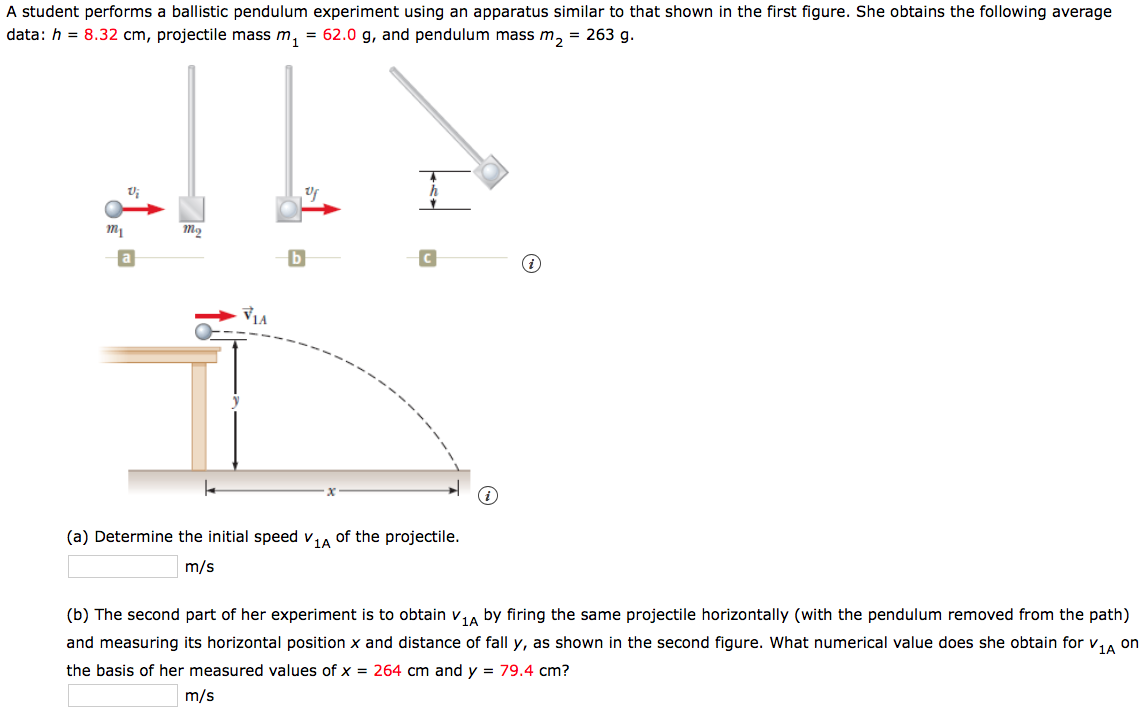# A student performs a ballistic pendulum experiment using an apparatus similar to that shown in the first figure. She obtains the following averagedata: h = 8.32 cm, projectile mass m, = 62.0 g, and pendulum mass m, = 263 g.VIAof the projectile.(a) Determine the initial speed v1Am/s(b) The second part of her experiment is to obtain v,A by firing the same projectile horizontally (with the pendulum removed from the path)and measuring its horizontal position x and distance of fall y, as shown in the second figure. What numerical value does she obtain for v,, onthe basis of her measured values of x = 264 cm and y = 79.4 cm?m/s

Question
58 viewshelp_outlineImage TranscriptioncloseA student performs a ballistic pendulum experiment using an apparatus similar to that shown in the first figure. She obtains the following average data: h = 8.32 cm, projectile mass m, = 62.0 g, and pendulum mass m, = 263 g. VIA of the projectile. (a) Determine the initial speed v 1A m/s (b) The second part of her experiment is to obtain v,A by firing the same projectile horizontally (with the pendulum removed from the path) and measuring its horizontal position x and distance of fall y, as shown in the second figure. What numerical value does she obtain for v,, on the basis of her measured values of x = 264 cm and y = 79.4 cm? m/s fullscreen
check_circle

Step 1

(a)

From the conservation of linear momentum,

Step 2

Substituting the values gives

Step 3

(b)

From kinematic e...

### Want to see the full answer?

See Solution

#### Want to see this answer and more?

Solutions are written by subject experts who are available 24/7. Questions are typically answered within 1 hour.*

See Solution
*Response times may vary by subject and question.
Tagged in

### Physics